# Estimation of E-field strength at a distance from dipole antenna

• I
Bobymayor
Hello everyone,

I was asking myself about electric field strength estimation at a distance d from - in my case - a half wave dipole antenna.
There are pretty much a lot of information about this on internet or in books but still, there are a few things that are confusing to me that I would like you guys to help me out !

"Settings" :
- Frequency F = 2GHz
- wavelength Wv = 150 mm
- Length of antenna L = 71,25 mm (0.475*Wv)
- Feeder impedance 73 ohms
- Power input = 1W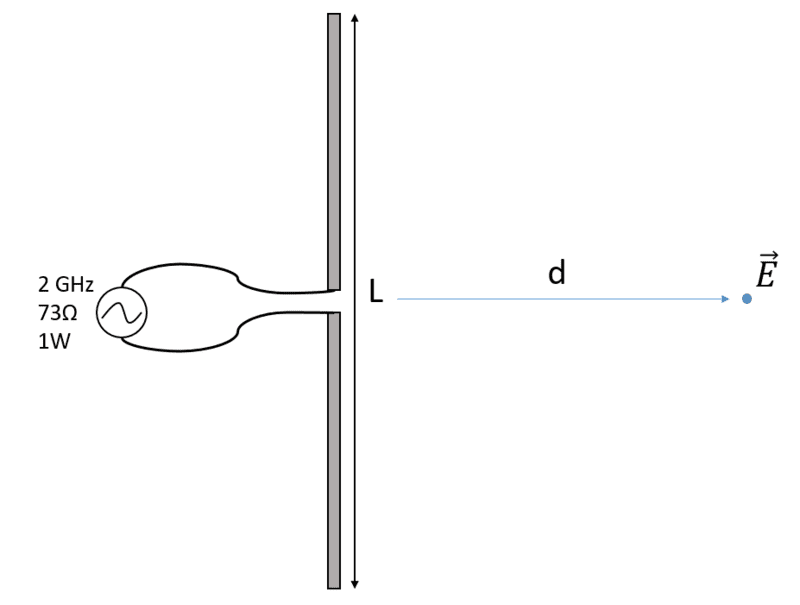It is said that for a halfwave dipole antenna, Electric field strength can be calculated from an approximation (in the case where d is orthogonal to L) which is :

I already have a problem here, isn't the electric field strengh supposed to decrease with square distance in far field, (and cube distance in near field) ??
Another source here (okay I admit, Wiki but I guess it's reliable sometimes) gives the following equation regarding radiated power :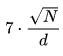Which again is decreasing proportionaly !

I simulated in an EM analysis software (CST) this half wave dipole antenna following standard design rules and same parameters (and in the void) and it works pretty accurately at 2 GHz. Plotting the E strength along the distance, from the antenna to 1000 mm (several times Wv), we can see the shape here :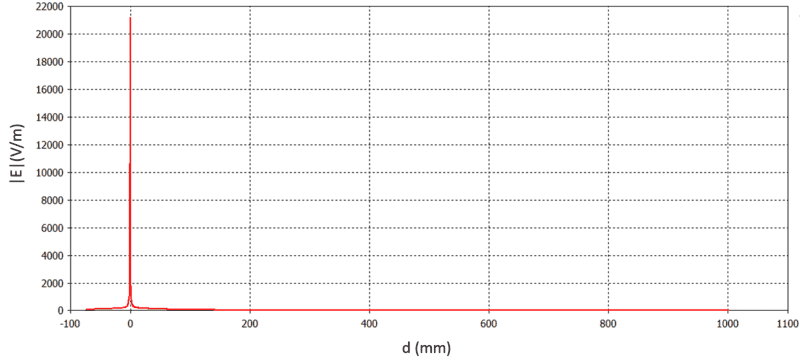If we zoom into remove the peak around 0 :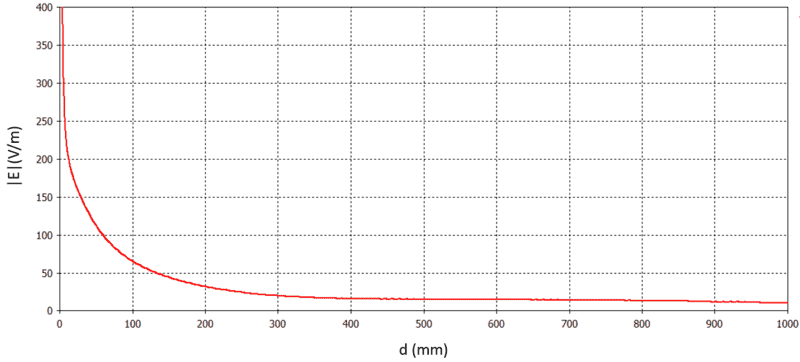And even more :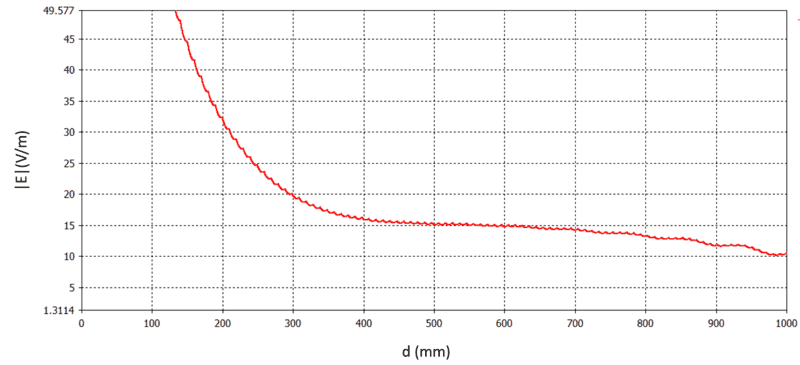There may be something obvious I missed ? These formulas are very big approximation because it's impossible to estimate it ? If you could enlighten me I would be very glad !

#### Attachments

•Delta2

Gold Member
The dipole in free space (no ground anywhere) radiates waves which fall in intensity with the inverse square law. By this I mean that the power/sq metre falls off with 1/D^2. The field components of this wave, electric and magnetic, fall inversely with distance, so for instance, E falls off with 1/D.

The formula you quote is correct for distances greater than about a sixth of a wavelength. E = (7x sqrt P)/D in Volts, metres and Watts. So the electric field strength falls off inversely with distance.

Now some more difficult information. At distances closer than about a sixth of a wavelength, we see some intense additional fields known as induction fields. Further, large antennas also have a region called the Near Radiation Zone where the pattern is distorted and it is possible for hot spots to occur. I think a dipole has a slight Near Radiation Zone and this may account for the slight hump seen around 600 mm. The distant pattern of a dipole resembles a ring doughnut, but is different when measured close up. Then we see a Near Radiation Zone, and the hole in the centre disappears.

If we approach the antenna in its equatorial plane, the electric field increases with 1/D until a distance of, say, lambda/2 from the antenna, then remains constant. As we almost touch the antenna we see an intense local field from the voltage across the feedpoint. If we similarly measure the magnetic field starting at D=lambda/2, it does not level off but continues upwards, following 1/D, until we touch the antenna conductor.

•DaveE
Bobymayor
Thank you for your answer, does this law differs depending on the antenna type (horn, V, tulip, monopolar,...) , if we consider always being in the maximum directivity axis for each of them ? What you explained is very clear and I understand that there are many parameters to consider, but I am sure somewhere, someday, I was taught that electric field decreases in D² in some case ! Maybe it is for static E-field..?

Homework Helper
Gold Member
The near field from a dipole antenna has ##\frac{1}{r^2}## and ##\frac{1}{r^3}## terms while the far field has ##\frac{1}{r}## term. This is demonstrated in any standard textbook about dipole antennas.